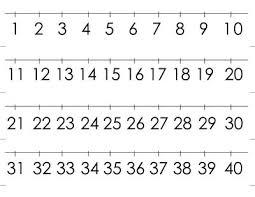# Consecutive 6090

The sum of four consecutive even numbers is equal to 108. Which of the numbers is equal to the smallest of these summands?

a =  24

### Step-by-step explanation:

a+(a+2)+(a+4)+(a+6)= 108

4a = 96

a = 96/4 = 24

a = 24

Our simple equation calculator calculates it.Did you find an error or inaccuracy? Feel free to write us. Thank you!

Tips for related online calculators
Do you have a linear equation or system of equations and looking for its solution? Or do you have a quadratic equation?